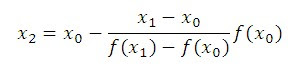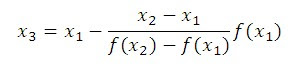# False Position (Regula falsi) Method Matlab Program, Algorithm & Flowchart

The false position method or regula falsi method is a term for problem-solving methods in arithmetic, algebra, and calculus. In simple terms, these methods begin by attempting to evaluate a problem using test ("false") values for the variables, and then adjust the values accordingly.

False Position method is the oldest method for finding the real roots of an equation f(x)=0. Also, this method closely resembles with Bisection method.

In False Position method, we choose two points x0 and x1, such that f(x0) and f(x1) are of opposite sign. So the abscissa of point where the chords cuts the x-axis (y=0) is given by,This is the first approximation to the root.

If now f(x0) and f(x2) are of opposite sign then the root lies between x0 and x2, so by replacing x1 by x2.

By using the following equation we obtain the second approximation:Similarly, we can obtain next approximations using same equations as above. After certain approximations (when two consecutive approximations are almost same) we will get our final answer. At the end of this article, we will see how to write MATLAB program for the False Position method.

Let us solve one simple problem for clear understanding.

## Example

Find the real root of the equation  x^3-2x-5=0 by using false position method.

## Solution

Let f(x) = x^3-2x-5

• F(2) = -1 = negative

• F(3) = 16 = positive

Hence the roots of given equation lies between (2,3)

Let us find first approximation (x2) by taking x0=2, x1=3,

X2 = x0 – {(x1 – x0)/[f(x1) – f(x0)]}*f(x0)

Therefore, x2 = 2 – [(3-2)/(16+1)]*(-1) = 2.0588

Here, f(x2) = f(2.0588) = -0.3908 = negative

Hence, roots of given equation lies between (2.0588,3)

X3 = x1 – {(x2 – x1)/[f(x2) – f(x1)]}*f(x1)

Therefore, x3 = 2.0588 – [(3-2.0588)/(16+0.3908)]*(-0.3908) = 2.0813

Here, f(x3) = f(2.0813) = -0.1469 = negative

Hence roots lies between (2.0813,3).

Therefore, x4=2.0896

Here, f(x4)=-0.0547

Hence root lies between (2.0896,3)

Therefore, x5=2.0927

Here, f(x5)=-0.0202

Hence root lies between (2.0927,3)

Therefore, x6=2.0939

Here, f(x6)=-0.0075

Hence root lies between (2.0939,3)

Therefore, x7=2.0943

Here, f(x8)=-0.0027

Hence root lies between (2.0943,3)

Therefore, x9=2.0945

Here, f(x9)=-0.0010

Hence root lies between (2.0945,3)

Therefore, x10=2.0945

Now, x9 and x10 are equal, therefore we can stop here.

So our final answer is x=2.0945.